# Setting the EEG reference#

This tutorial describes how to set or change the EEG reference in MNE-Python.

As usual we’ll start by importing the modules we need, loading some example data, and cropping it to save memory. Since this tutorial deals specifically with EEG, we’ll also restrict the dataset to just a few EEG channels so the plots are easier to see:

```import os
import mne

sample_data_folder = mne.datasets.sample.data_path()
sample_data_raw_file = os.path.join(sample_data_folder, 'MEG', 'sample',
'sample_audvis_raw.fif')
raw.pick(['EEG 0{:02}'.format(n) for n in range(41, 60)])
```
```Reading 0 ... 36037  =      0.000 ...    60.000 secs...
Removing projector <Projection | PCA-v1, active : False, n_channels : 102>
Removing projector <Projection | PCA-v2, active : False, n_channels : 102>
Removing projector <Projection | PCA-v3, active : False, n_channels : 102>
```
Measurement date December 03, 2002 19:01:10 GMT MEG Unknown 146 points 19 EEG EEG 053 Not available Not available 600.61 Hz 0.10 Hz 172.18 Hz sample_audvis_raw.fif 00:01:01 (HH:MM:SS)

## Background#

EEG measures a voltage (difference in electric potential) between each electrode and a reference electrode. This means that whatever signal is present at the reference electrode is effectively subtracted from all the measurement electrodes. Therefore, an ideal reference signal is one that captures none of the brain-specific fluctuations in electric potential, while capturing all of the environmental noise/interference that is being picked up by the measurement electrodes.

In practice, this means that the reference electrode is often placed in a location on the subject’s body and close to their head (so that any environmental interference affects the reference and measurement electrodes similarly) but as far away from the neural sources as possible (so that the reference signal doesn’t pick up brain-based fluctuations). Typical reference locations are the subject’s earlobe, nose, mastoid process, or collarbone. Each of these has advantages and disadvantages regarding how much brain signal it picks up (e.g., the mastoids pick up a fair amount compared to the others), and regarding the environmental noise it picks up (e.g., earlobe electrodes may shift easily, and have signals more similar to electrodes on the same side of the head).

Even in cases where no electrode is specifically designated as the reference, EEG recording hardware will still treat one of the scalp electrodes as the reference, and the recording software may or may not display it to you (it might appear as a completely flat channel, or the software might subtract out the average of all signals before displaying, making it look like there is no reference).

## Setting or changing the reference channel#

If you want to recompute your data with a different reference than was used when the raw data were recorded and/or saved, MNE-Python provides the `set_eeg_reference()` method on `Raw` objects as well as the `mne.add_reference_channels()` function. To use an existing channel as the new reference, use the `set_eeg_reference()` method; you can also designate multiple existing electrodes as reference channels, as is sometimes done with mastoid references:

```# code lines below are commented out because the sample data doesn't have
# earlobe or mastoid channels, so this is just for demonstration purposes:

# use a single channel reference (left earlobe)
# raw.set_eeg_reference(ref_channels=['A1'])

# use average of mastoid channels as reference
# raw.set_eeg_reference(ref_channels=['M1', 'M2'])

# use a bipolar reference (contralateral)
# raw.set_bipolar_reference(anode='[F3'], cathode=['F4'])
```

If a scalp electrode was used as reference but was not saved alongside the raw data (reference channels often aren’t), you may wish to add it back to the dataset before re-referencing. For example, if your EEG system recorded with channel `Fp1` as the reference but did not include `Fp1` in the data file, using `set_eeg_reference()` to set (say) `Cz` as the new reference will then subtract out the signal at `Cz` without restoring the signal at `Fp1`. In this situation, you can add back `Fp1` as a flat channel prior to re-referencing using `add_reference_channels()`. (Since our example data doesn’t use the 10-20 electrode naming system, the example below adds `EEG 999` as the missing reference, then sets the reference to `EEG 050`.) Here’s how the data looks in its original state:

```raw.plot()
```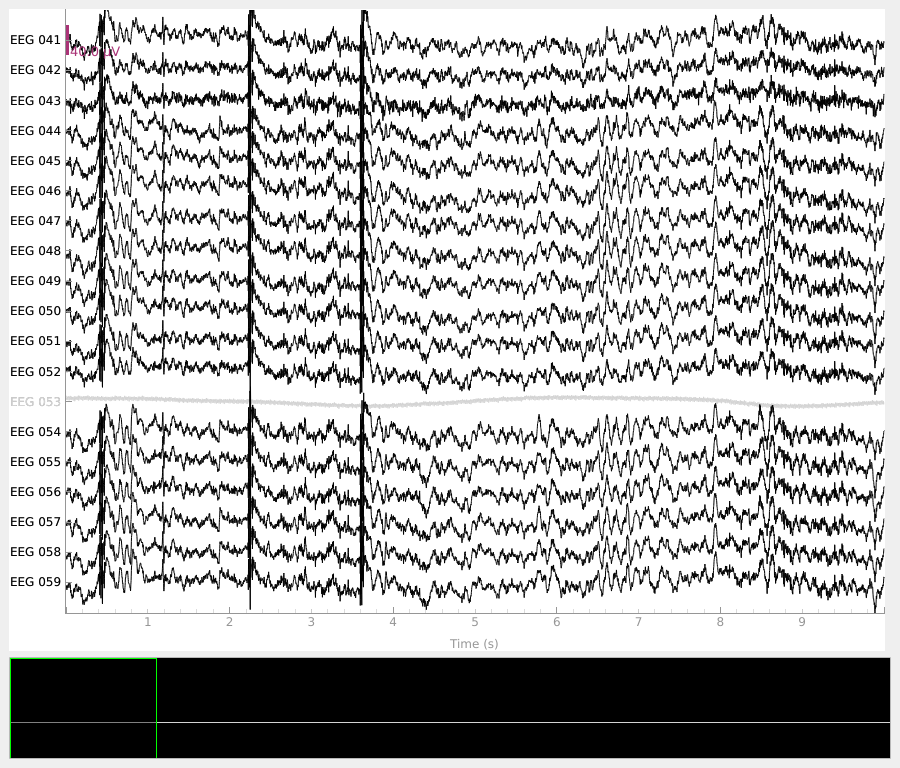By default, `add_reference_channels()` returns a copy, so we can go back to our original `raw` object later. If you wanted to alter the existing `Raw` object in-place you could specify `copy=False`.

```# add new reference channel (all zero)
raw_new_ref.plot()
```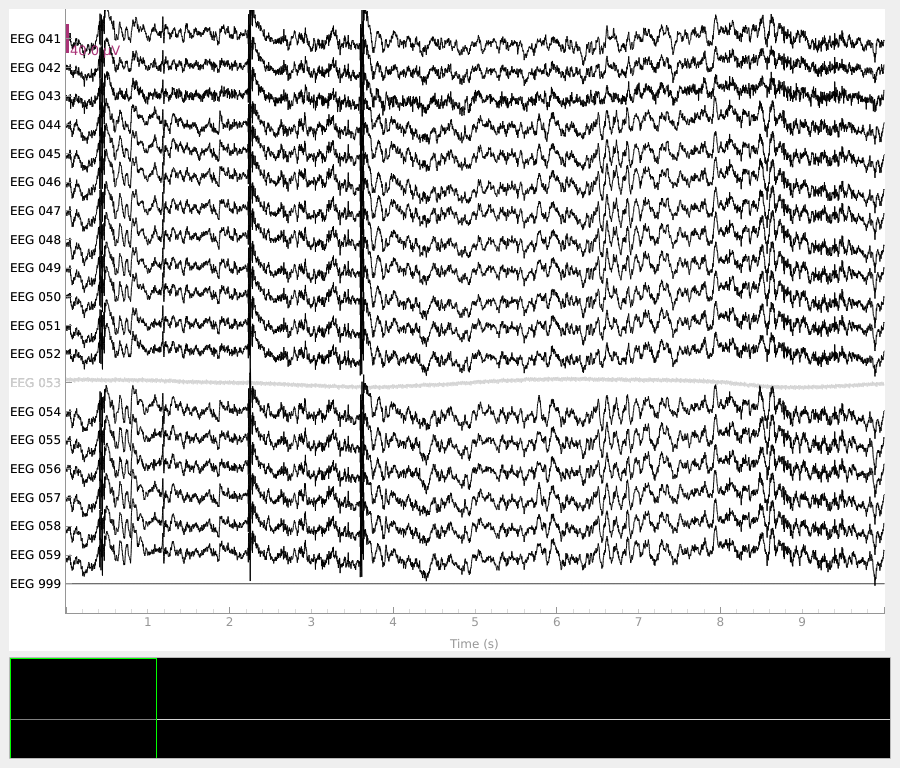```# set reference to `EEG 050`
raw_new_ref.set_eeg_reference(ref_channels=['EEG 050'])
raw_new_ref.plot()
```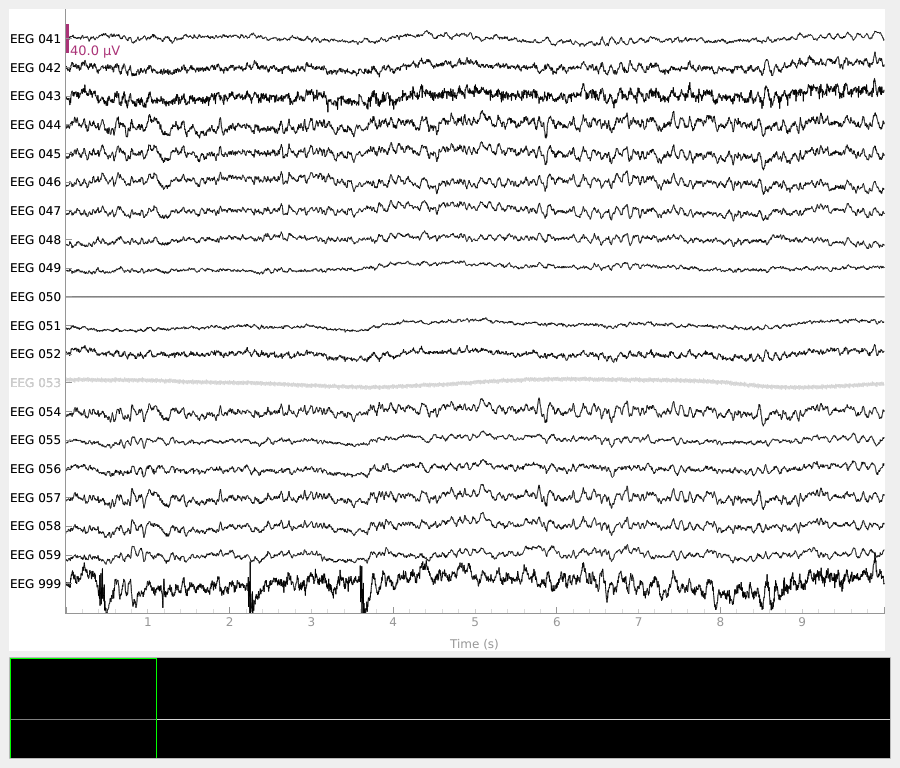```EEG channel type selected for re-referencing
Applying a custom ('EEG',) reference.
```

Notice that the new reference (`EEG 050`) is now flat, while the original reference channel that we added back to the data (`EEG 999`) has a non-zero signal. Notice also that `EEG 053` (which is marked as “bad” in `raw.info['bads']`) is not affected by the re-referencing.

## Setting average reference#

To set a “virtual reference” that is the average of all channels, you can use `set_eeg_reference()` with `ref_channels='average'`. Just as above, this will not affect any channels marked as “bad”, nor will it include bad channels when computing the average. However, it does modify the `Raw` object in-place, so we’ll make a copy first so we can still go back to the unmodified `Raw` object later:

```# use the average of all channels as reference
raw_avg_ref = raw.copy().set_eeg_reference(ref_channels='average')
raw_avg_ref.plot()
```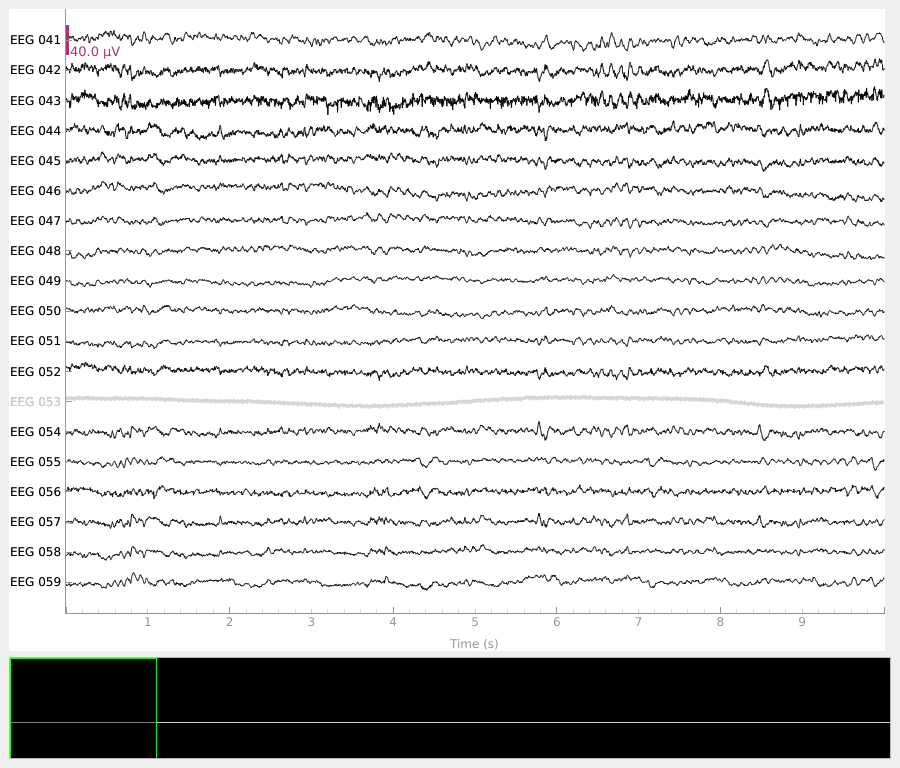```EEG channel type selected for re-referencing
Applying average reference.
Applying a custom ('EEG',) reference.
```

## Creating the average reference as a projector#

If using an average reference, it is possible to create the reference as a projector rather than subtracting the reference from the data immediately by specifying `projection=True`:

```raw.set_eeg_reference('average', projection=True)
print(raw.info['projs'])
```
```EEG channel type selected for re-referencing
1 projection items deactivated
Average reference projection was added, but has not been applied yet. Use the apply_proj method to apply it.
[<Projection | Average EEG reference, active : False, n_channels : 18>]
```

Creating the average reference as a projector has a few advantages:

1. It is possible to turn projectors on or off when plotting, so it is easy to visualize the effect that the average reference has on the data.

2. If additional channels are marked as “bad” or if a subset of channels are later selected, the projector will be re-computed to take these changes into account (thus guaranteeing that the signal is zero-mean).

3. If there are other unapplied projectors affecting the EEG channels (such as SSP projectors for removing heartbeat or blink artifacts), EEG re-referencing cannot be performed until those projectors are either applied or removed; adding the EEG reference as a projector is not subject to that constraint. (The reason this wasn’t a problem when we applied the non-projector average reference to `raw_avg_ref` above is that the empty-room projectors included in the sample data `.fif` file were only computed for the magnetometers.)

```for title, proj in zip(['Original', 'Average'], [False, True]):
with mne.viz.use_browser_backend('matplotlib'):
fig = raw.plot(proj=proj, n_channels=len(raw))
# make room for title
fig.suptitle('{} reference'.format(title), size='xx-large', weight='bold')
```
•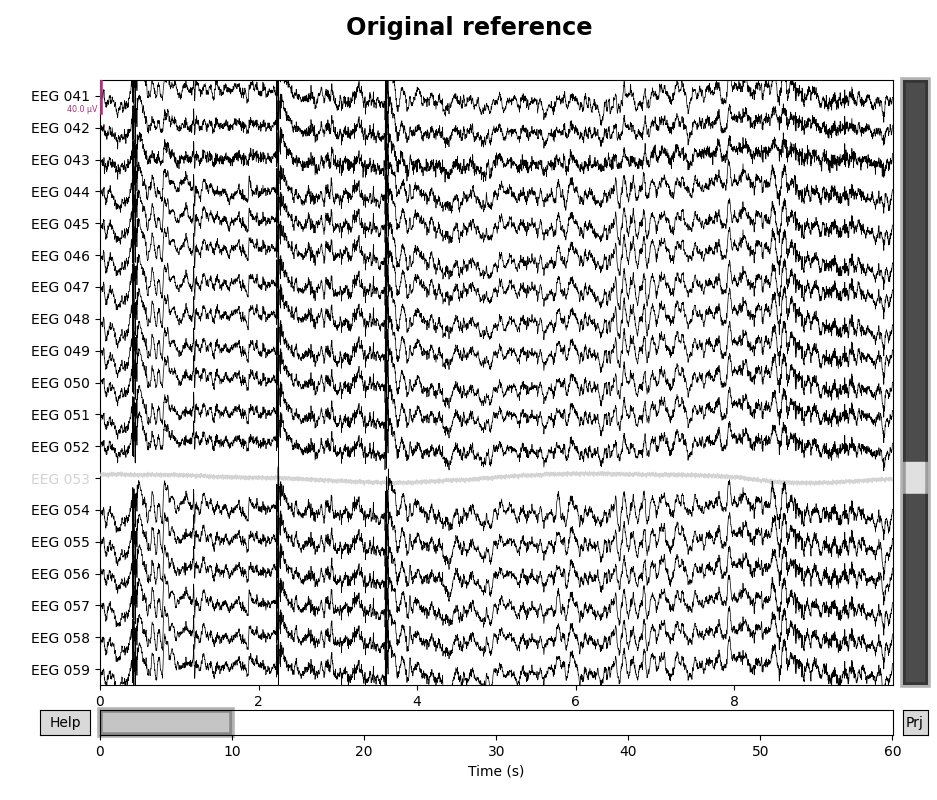•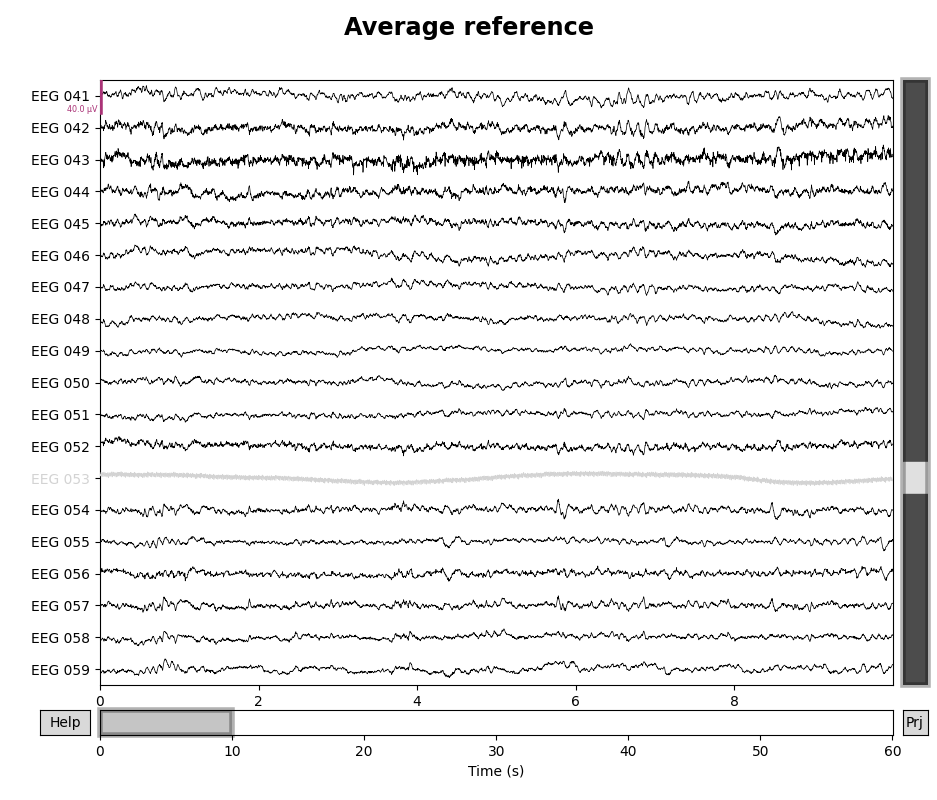```Using matplotlib as 2D backend.
Using qt as 2D backend.
Using matplotlib as 2D backend.
Using qt as 2D backend.
```

## Using an infinite reference (REST)#

To use the “point at infinity” reference technique described in  requires a forward model, which we can create in a few steps. Here we use a fairly large spacing of vertices (`pos` = 15 mm) to reduce computation time; a 5 mm spacing is more typical for real data analysis:

```raw.del_proj()  # remove our average reference projector first
sphere = mne.make_sphere_model('auto', 'auto', raw.info)
src = mne.setup_volume_source_space(sphere=sphere, exclude=30., pos=15.)
forward = mne.make_forward_solution(raw.info, trans=None, src=src, bem=sphere)
raw_rest = raw.copy().set_eeg_reference('REST', forward=forward)

for title, _raw in zip(['Original', 'REST (∞)'], [raw, raw_rest]):
with mne.viz.use_browser_backend('matplotlib'):
fig = _raw.plot(n_channels=len(raw), scalings=dict(eeg=5e-5))
# make room for title
fig.suptitle('{} reference'.format(title), size='xx-large', weight='bold')
```
•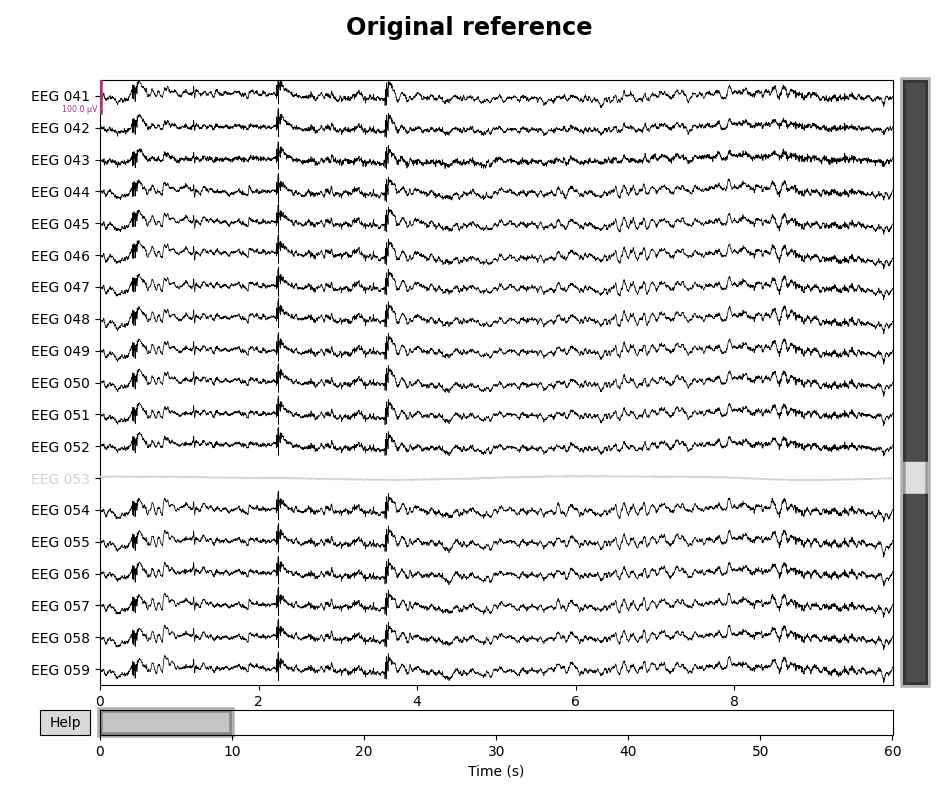•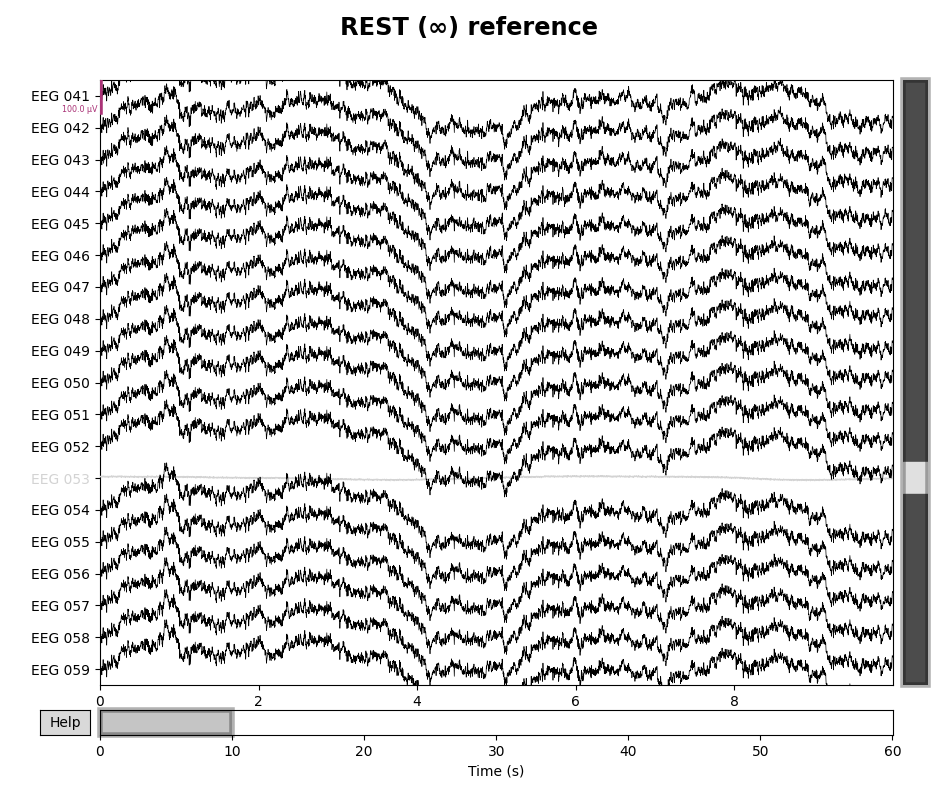```Fitted sphere radius:         91.0 mm
Origin head coordinates:      -4.1 16.0 51.7 mm
Origin device coordinates:    1.4 17.8 -10.3 mm

Equiv. model fitting -> RV = 0.00349057 %
mu1 = 0.944702    lambda1 = 0.137194
mu2 = 0.667435    lambda2 = 0.683781
mu3 = -0.26548    lambda3 = -0.0106058
Set up EEG sphere model with scalp radius    91.0 mm

Sphere                : origin at (-4.1 16.0 51.7) mm
grid                  : 15.0 mm
mindist               : 5.0 mm
Exclude               : 30.0 mm

Setting up the sphere...
Surface CM = (  -4.1   16.0   51.7) mm
Surface fits inside a sphere with radius   81.9 mm
Surface extent:
x =  -86.0 ...   77.8 mm
y =  -65.9 ...   97.9 mm
z =  -30.2 ...  133.7 mm
Grid extent:
x =  -90.0 ...   90.0 mm
y =  -75.0 ...  105.0 mm
z =  -45.0 ...  135.0 mm
2197 sources before omitting any.
655 sources after omitting infeasible sources not within 30.0 - 81.9 mm.
532 sources remaining after excluding the sources outside the surface and less than    5.0 mm inside.
Source space : MRI voxel -> MRI (surface RAS)
0.015000  0.000000  0.000000     -90.00 mm
0.000000  0.015000  0.000000     -75.00 mm
0.000000  0.000000  0.015000     -45.00 mm
0.000000  0.000000  0.000000       1.00
Source space          : <SourceSpaces: [<discrete, n_used=532>] MRI (surface RAS) coords, ~560 kB>
MRI -> head transform : identity
Measurement data      : instance of Info
Sphere model      : origin at [-0.00413199  0.0159844   0.05174612] mm
Standard field computations
Free source orientations

Read 1 source spaces a total of 532 active source locations

Coordinate transformation: MRI (surface RAS) -> head
1.000000  0.000000  0.000000       0.00 mm
0.000000  1.000000  0.000000       0.00 mm
0.000000  0.000000  1.000000       0.00 mm
0.000000  0.000000  0.000000       1.00

Read  19 EEG channels from info
Source spaces are now in head coordinates.

Using the sphere model.

Using the equivalent source approach in the homogeneous sphere for EEG
Computing EEG at 532 source locations (free orientations)...
[Parallel(n_jobs=1)]: Using backend SequentialBackend with 1 concurrent workers.
[Parallel(n_jobs=1)]: Done   1 out of   1 | elapsed:    0.3s remaining:    0.0s
[Parallel(n_jobs=1)]: Done   1 out of   1 | elapsed:    0.3s finished

Finished.
EEG channel type selected for re-referencing
Applying REST reference.
Applying a custom ('EEG',) reference.
18 out of 19 channels remain after picking
Using matplotlib as 2D backend.
Using qt as 2D backend.
Using matplotlib as 2D backend.
Using qt as 2D backend.
```

## Using a bipolar reference#

To create a bipolar reference, you can use `set_bipolar_reference()` along with the respective channel names for `anode` and `cathode` which creates a new virtual channel that takes the difference between two specified channels (anode and cathode) and drops the original channels by default. The new virtual channel will be annotated with the channel info of the anode with location set to `(0, 0, 0)` and coil type set to `EEG_BIPOLAR` by default. Here we use a contralateral/transverse bipolar reference between channels `EEG 054` and `EEG 055` as described in  which creates a new virtual channel named `EEG 054-EEG 055`.

```raw_bip_ref = mne.set_bipolar_reference(raw, anode=['EEG 054'],
cathode=['EEG 055'])
raw_bip_ref.plot()
```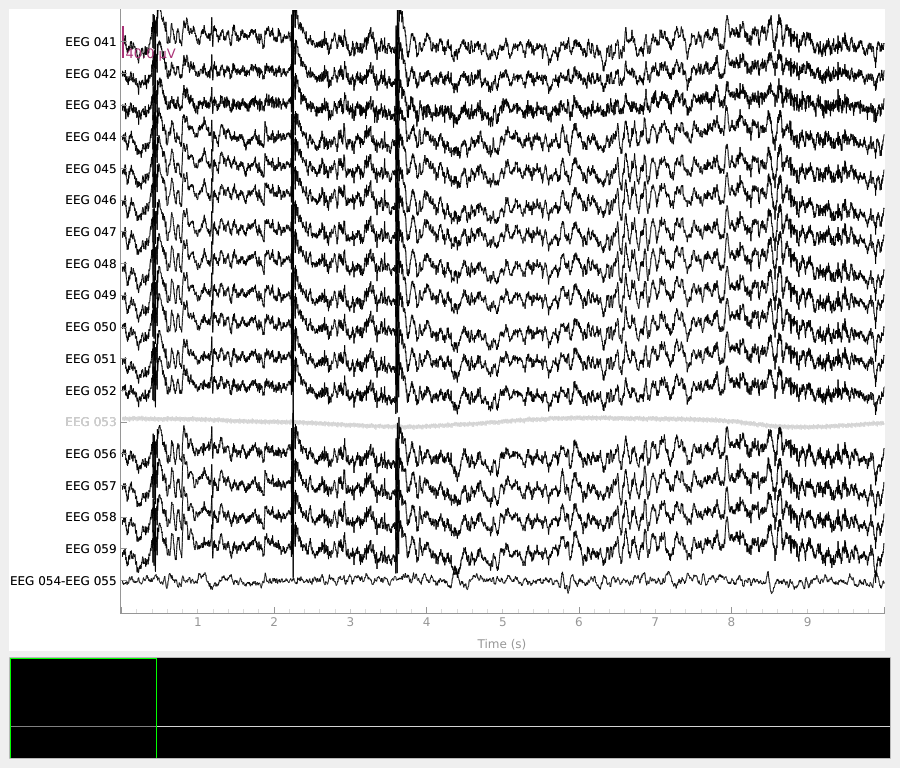```EEG channel type selected for re-referencing
Creating RawArray with float64 data, n_channels=1, n_times=36038
Range : 25800 ... 61837 =     42.956 ...   102.956 secs